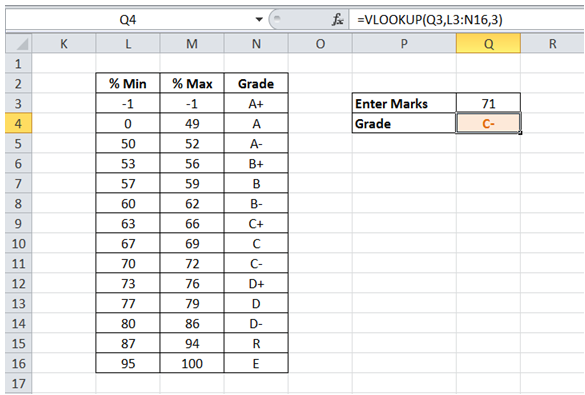# Excel Made Easy

## Slab where value fits range in Excel

For example, I want to get the grade given marks secured and grade definition Table.

To do it in Excel, here is the answer:

a) Enter the formula =VLOOKUP(Q3,L3:N16,3) where L3:N16 has grade definition slabs, Q3 represents the marks secured and 3 represents the column no. which has the desired results (Grade in this case).